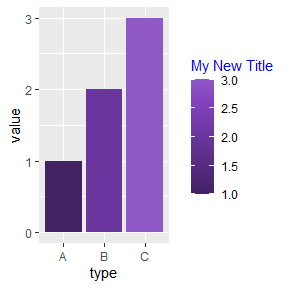# Using with {ggplot2}

Install from CRAN using:

install.packages("PrettyCols")

Or install the development version using:

remotes::install_github("nrennie/PrettyCols")

library(PrettyCols)

Set up a simple data frame to use for an example plot:

library(ggplot2)
df <- data.frame(type = c("A", "B", "C"),
value = c(1, 2, 3))

To change the fill or colour for discrete variables:

ggplot(data = df,
mapping = aes(x = type, y = value, fill = type)) +
geom_col() +
scale_fill_pretty_d(name = "Blues")

ggplot(data = df,
mapping = aes(x = type, y = value, colour = type)) +
geom_col(size = 2, fill = "white") +
scale_colour_pretty_d(name = "Blues")
#> Warning: Using size aesthetic for lines was deprecated in ggplot2 3.4.0.
#> ℹ Please use linewidth instead.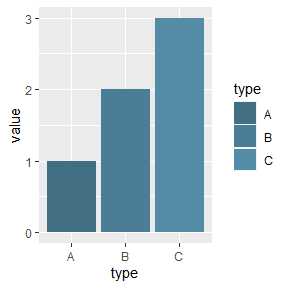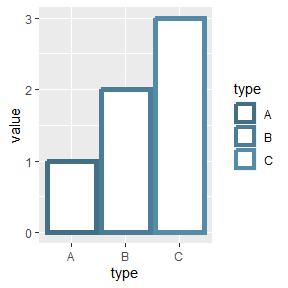To change the fill or colour for continuous variables:

ggplot(data = df,
mapping = aes(x = type, y = value, fill = value)) +
geom_col() +
scale_fill_pretty_c(name = "Purples")

ggplot(data = df,
mapping = aes(x = type, y = value, colour = value)) +
geom_col(size = 2, fill = "white") +
scale_colour_pretty_c(name = "Purples", direction = -1)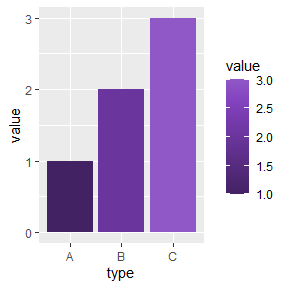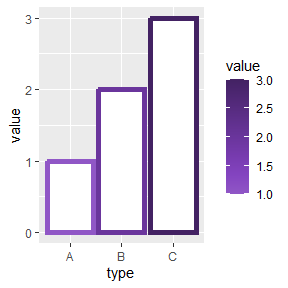To change the legend title:

ggplot(data = df,
mapping = aes(x = type, y = value, fill = value)) +
geom_col() +
scale_fill_pretty_c(name = "Purples", legend_title = "My New Title") +
theme(legend.title = element_text(colour = "blue"))